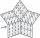# Supermarket 2

A supermarket had a buko pie sale. In the morning 2/3 of the pies were sold and in the afternoon 1/6 of the pies were sold. If 150 pies were left, how many pies had been sold? Show your solution.

Result

x =  750

#### Solution:

x + 150 = n
x = 2/3 n + 1/6 n

n-x = 150
5n-6x = 0

n = 900
x = 750

Calculated by our linear equations calculator.

Leave us a comment of example and its solution (i.e. if it is still somewhat unclear...):

Showing 0 comments:Be the first to comment!#### To solve this example are needed these knowledge from mathematics:

Need help calculate sum, simplify or multiply fractions? Try our fraction calculator. Do you have a linear equation or system of equations and looking for its solution? Or do you have quadratic equation?

## Next similar examples:

1. Hotel roomsIn the 45 rooms, there were 169 guests, some rooms were three-bedrooms and some five-bedrooms. How many rooms were?
2. Simple equation 6Solve equation with one variable: X/2+X/3+X/4=X+4
3. ATC campThe owner of the campsite offers 79 places in 22 cabins. How many of them are triple and quadruple?
4. Football seasonDalibor and Adam together scored 97 goals in the season. Adam scored 9 goals more than Dalibor. How many goals scored each?
5. Fifth of the numberThe fifth of the number is by 24 less than that number. What is the number?
6. PC disksPeter has 45 disks in three colors. One-fifth of the disks are blue, red are twice more than the white. How much is blue, red and white disks?
7. Three brothersThe three brothers have a total of 42 years. Jan is five years younger than Peter and Peter is 2 years younger than Michael. How many years has each of them?
8. SchoolsThree schools are attended by 678 pupils. To the first attend 21 students more and to the third 108 fewer students than to second school. How many students attend the schools?
9. Equations - simpleSolve system of linear equations: x-2y=6 3x+2y=4
10. Two numbersThe sum of the two numbers is 1. Find both numbers if you know that half of the first is equal to one seventh of the second number.
11. BlackberriesDaniel, Jolana and Stano collected together 34 blackberries. Daniel collected 8 blackberries more than Jolana, Jolana 4 more than Stano. Determine the number blackberries each collected .
12. Three figures - numbersThe sum of three numbers, if each is 10% larger than the previous one, is 662. Determine the figures.
13. Value 4If 5/18=425 what is the value 13/18?
14. Rabbits 3Viju has 40 chickens and rabbits. If in all there are 90 legs. How many rabbits are there with Viju?
15. Unknown number 23Find 2/3 of unknown number, which is two thirds of the 99.
16. Linear systemSolve this linear system (two linear equations with two unknowns): x+y =36 19x+22y=720
17. Vijuviju has 40 chickens and rabbits. If in all there are 90 legs. How many rabbits are there with viju??